Ex 3.2

Chapter 3 Class 12 Matrices
Serial order wise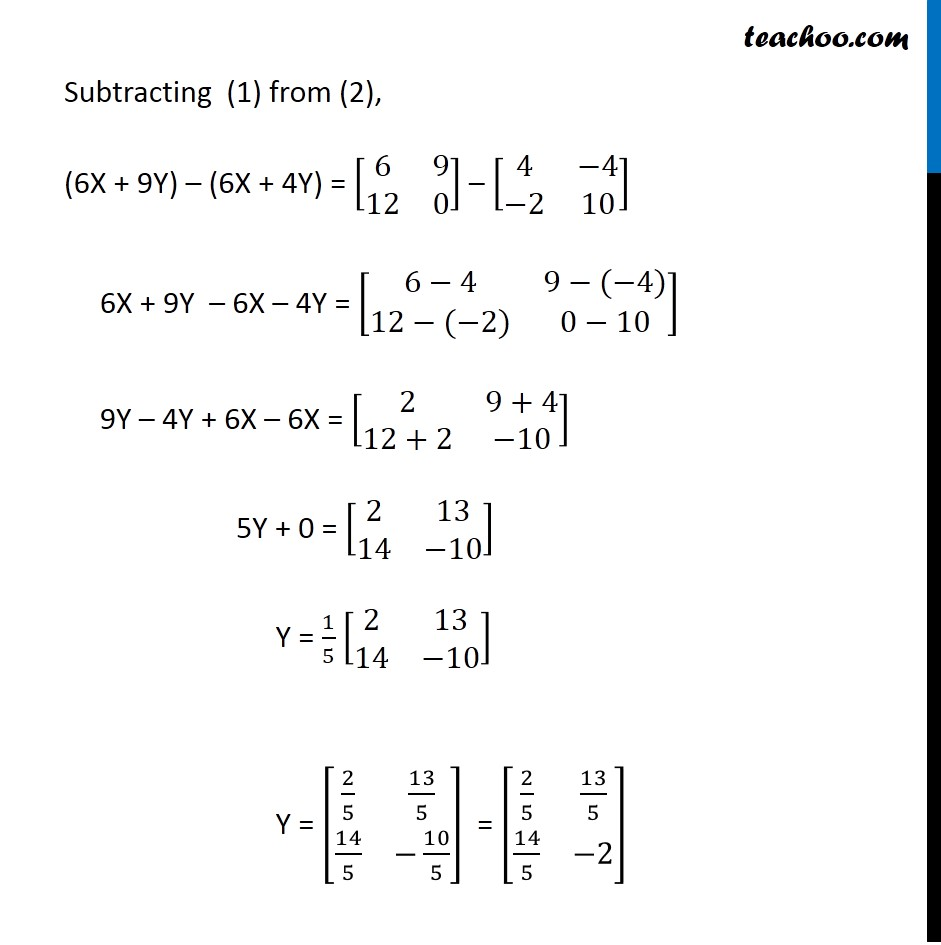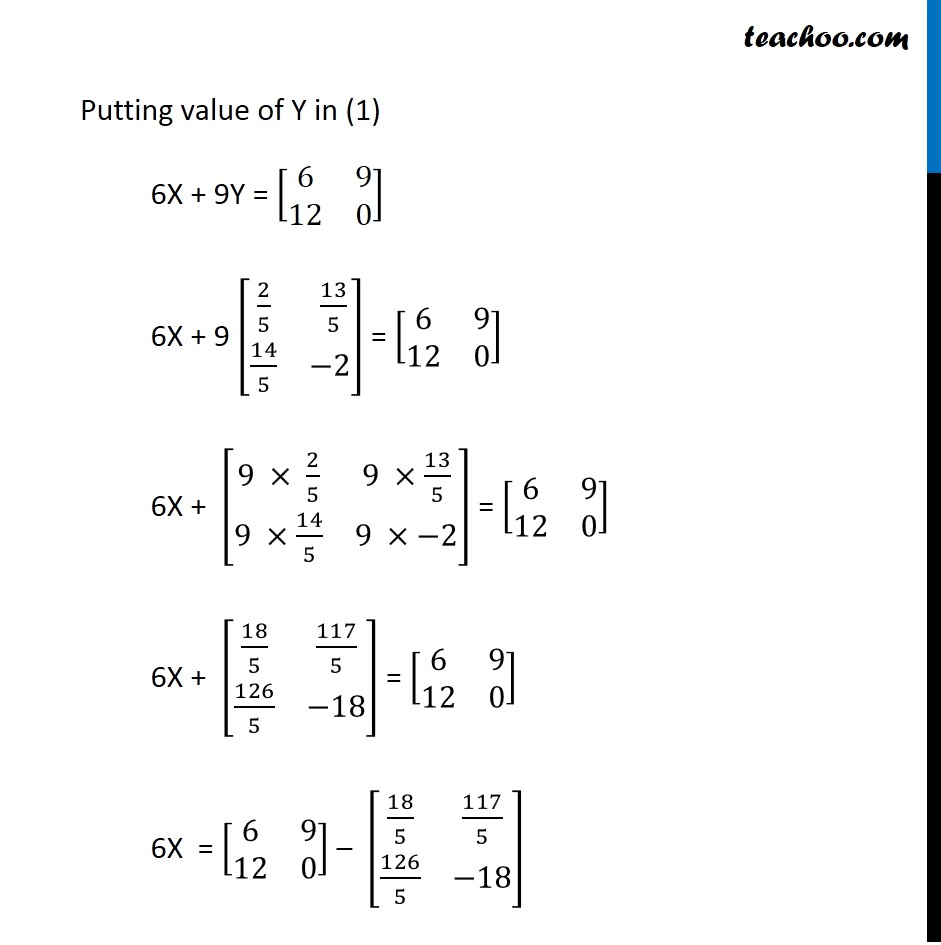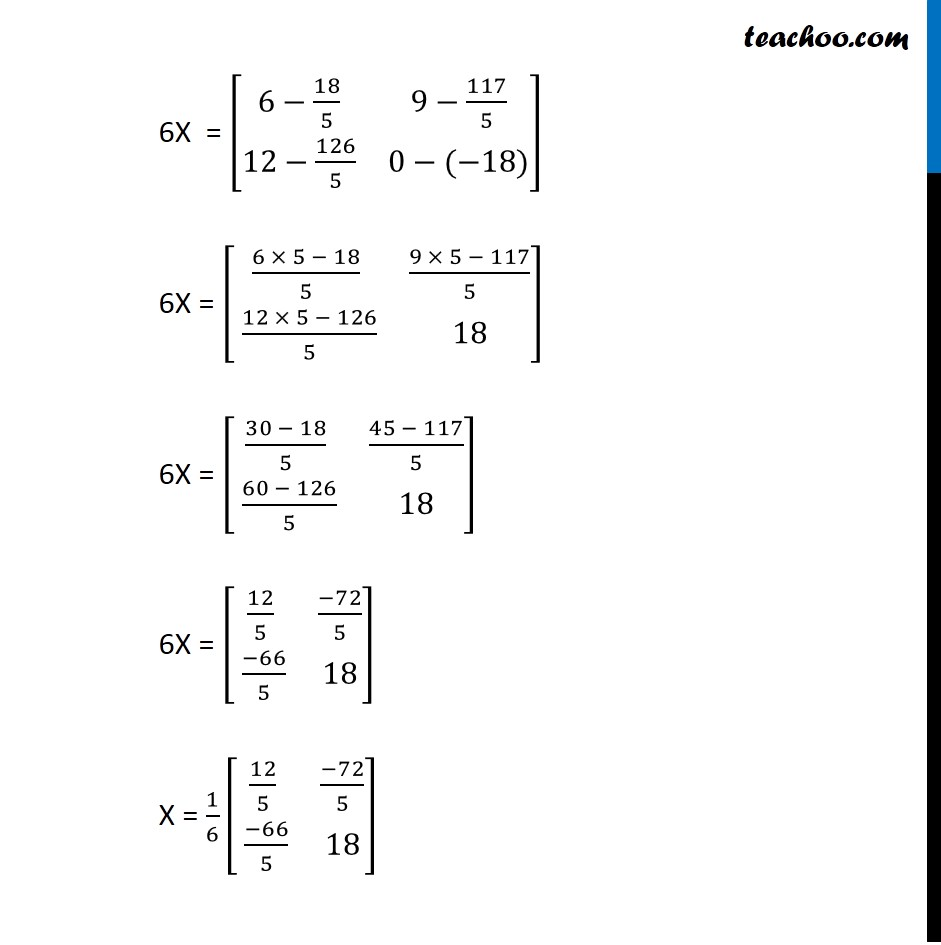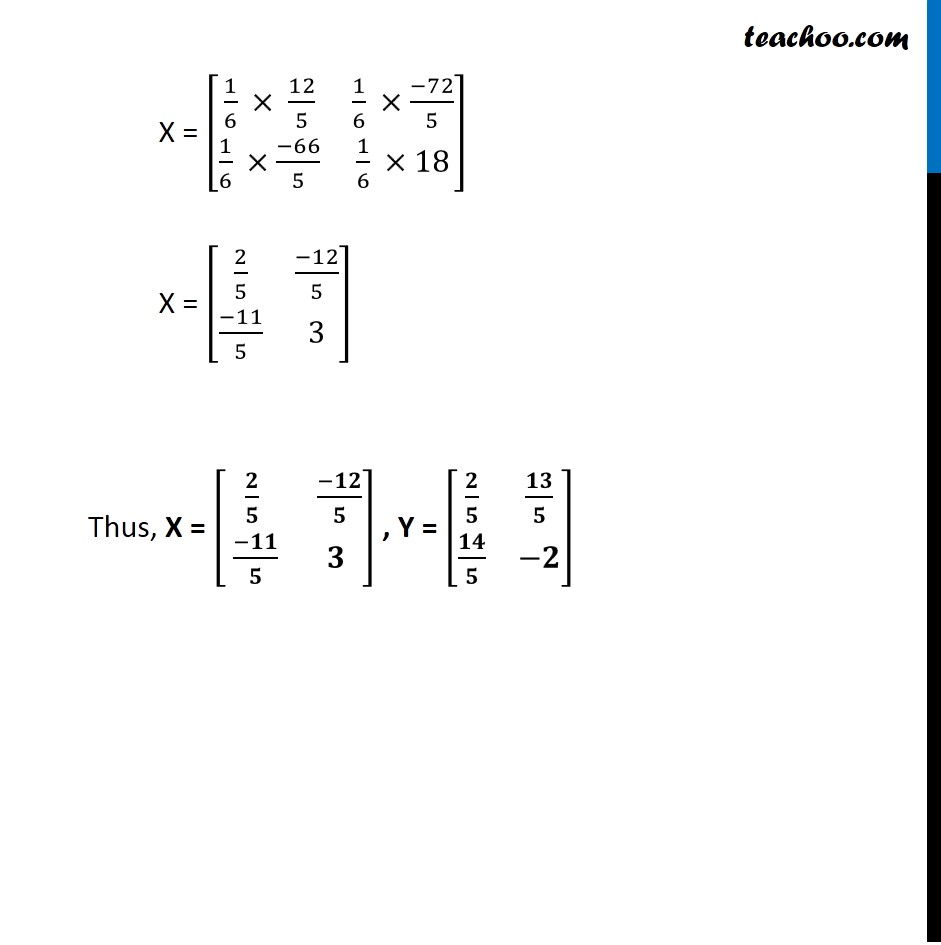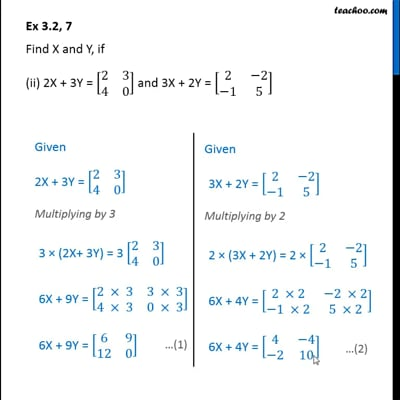This video is only available for Teachoo black users

Maths Crash Course - Live lectures + all videos + Real time Doubt solving!

### Transcript

Ex 3.2, 7 Find X and Y, if (ii) 2X + 3Y = [■8(2&[email protected]&0)] and 3X + 2Y = [■8(2&−[email protected]−1&5)] Given 2X + 3Y = [■8(2&[email protected]&0)] Multiplying by 3 3 × (2X+ 3Y) = 3 [■8(2&[email protected]&0)] 6X + 9Y = [■8(2 × 3&3 × [email protected] × 3&0 × 3)] 6X + 9Y = [■8(6&[email protected]&0)] Given 3X + 2Y = [■8(2&−[email protected]−1&5)] Multiplying by 2 2 × (3X + 2Y) = 2 × [■8(2&−[email protected]−1&5)] 6X + 4Y = [■8(2 ×2&−2 ×[email protected]−1 ×2&5 ×2)] 6X + 4Y = [■8(4&−[email protected]−2&10)] Subtracting (1) from (2), (6X + 9Y) – (6X + 4Y) = [■8(6&[email protected]&0)] – [■8(4&−[email protected]−2&10)] 6X + 9Y – 6X – 4Y = [■8(6−4&9−(−4)@12−(−2)&0−10)] 9Y – 4Y + 6X – 6X = [■8(2&[email protected]+2&−10)] 5Y + 0 = [■8(2&[email protected]&−10)] Y = 1/5 [■8(2&[email protected]&−10)] Y = [■8(2/5&13/[email protected]/5&−10/5)] = [■8(2/5&13/[email protected]/5&−2)] Putting value of Y in (1) 6X + 9Y = [■8(6&[email protected]&0)] 6X + 9 [■8(2/5& 13/[email protected]/5&−2)] = [■8(6&[email protected]&0)] 6X + [■8(9 × 2/5&9 ×13/[email protected] ×14/5&9 ×−2)] = [■8(6&[email protected]&0)] 6X + [■8(18/5&117/[email protected]/5&−18)] = [■8(6&[email protected]&0)] 6X = [■8(6&[email protected]&0)] – [■8(18/5&117/[email protected]/5&−18)] 6X = [■8(6−18/5&9−117/[email protected]−126/5&0−(−18))] 6X = [■8((6 × 5 − 18)/5&(9 × 5 − 117)/[email protected] (12 × 5 − 126)/5&18)] 6X = [■8((30 − 18)/5&(45 − 117)/[email protected] (60 − 126)/5&18)] 6X = [■8(12/5&(−72)/[email protected] (−66)/5&18)] X = 1/6 [■8(12/5&(−72)/[email protected] (−66)/5&18)] X = [■8(1/6 × 12/5&1/6 ×(−72)/[email protected]/6 ×(−66)/5&1/6 ×18)] X = [■8(2/5&(−12)/[email protected](−11)/5&3)] Thus, X = [■8(𝟐/𝟓& (−𝟏𝟐)/𝟓@ (−𝟏𝟏)/𝟓&𝟑)] , Y = [■8(𝟐/𝟓&𝟏𝟑/𝟓@𝟏𝟒/𝟓&−𝟐)]